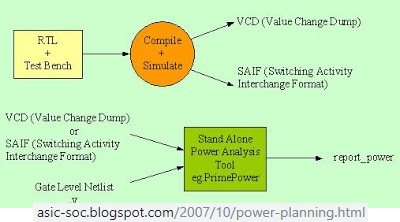# Impact of Input Toggling on the Power for Different MAC Implementations

A deep neural network (DNN) is an artificial neural network (ANN) with multiple layers between the input and output layers. The DNN finds the correct mathematical manipulation to turn the input into the output, whether it be a linear relationship or a non-linear relationship.Multiply-and-accumulate (MAC) operations are the core of deep neural networks (DNNs). In this project we would like to investigate to what extent the toggling of the MAC unit inputs impacts its energy consumption.Project description:

A deep neural network (DNN) is an artificial neural network (ANN) with multiple layers between the input and output layers. The DNN finds the correct mathematical manipulation to turn the input into the output, whether it be a linear relationship or a non-linear relationship.

Multiply-and-accumulate (MAC) operations are the core of deep neural networks (DNNs). In this project we would like to investigate to what extent the toggling of the MAC unit inputs impacts its energy consumption.

In this project the students will:

1) Explore the different MAC implementations.

2) Implement them in SystemVerilog.

3) Run dynamic power simulations with different input variations.

4) Evaluate the amount of toggling in a real DNN.